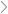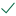Questions & AnswersMicroeconomicsYou have \$100 to invest in one of two assets.    The first asset,...

QuestionAnswered step-by-step

# You have \$100 to invest in one of two assets.    The first asset,...

You have \$100 to invest in one of two assets.

The first asset, called Asset A, is a traditional asset.  You receive a 5% return for every dollar you invest.

The second asset, called Asset B, is more complicated.  To calculate the return on Asset B we will sum up all the funds invested in Asset B, multiply by 10% and divide by the total number of people in the class.

An example: suppose there are 20 people involved in this exercise.  Suppose that you invest \$100 in Asset A and assume that there is a total of \$1,000 invested in Asset B.  Then your return would be equal to:

.05(\$100) + .1(\$1,000)/20 = \$5 + \$5 = \$10

So everyone receives the same amount from Asset B regardless of how much someone invests in Asset B.  (For example, suppose Carol was in the class and she put all of her funds into Asset B. Her return would be .05(\$0) +.1(\$1,000/20) = \$0 + \$5 = \$5

Prove that no matter what others are doing that you are always individually better off if you invest all of your funds in Asset A

If the choice is to invest all in A or all in B prove that everyone is better off investing all in Asset B.

How Much Will You Invest in Asset A:

How Much Will You Invest in Asset B:Solved by verified expert
<p><strong>sectetur adipiscing elit. Nam lacinia pulvinar t</strong>sectetur adipiscing elit. Nam lacinia pulvinar tortor nec facilisis. Pellentesque dapibus efficitur laoreet. Nam risus ante, dapibus a molestie consequat, ultrices ac magna. Fusce dui lectus, congue vel laoreet ac, d</p>sectetur adipiscing elit. Nam lacinia10,000 step-by-step explanations